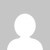# Python set operations | Basics with example code

Python Sets have mathematical set operations like union, intersection, difference, and symmetric difference. You can do this operation using the operators or inbuilt methods.

See below Operator for set operations:

• | for union
• & for intersection
• – for difference
• ^ for symmetric difference

## Python set operations examples

Simple example code.

### Set Union, S1|S2 operation

Union is performed using | operator or using the union() method.

``````fib = {1, 1, 2, 3, 5, 8}
prime = {2, 3, 5, 7, 11}

print(fib | prime)

# or using method
res = fib.union(prime)
print(res)``````

Output: {1, 2, 3, 5, 7, 8, 11}

### Set Intersection, S1&S2 operation

The intersection is performed using & operator using the intersection() method.

``````fib = {1, 1, 2, 3, 5, 8}
prime = {2, 3, 5, 7, 11}

print(fib & prime)

# or using method
res = fib.intersection(prime)
print(res)``````

Output: {2, 3, 5}

### Set Difference, S1-S2operation

The difference is performed using the – operator or using the difference() method.

``````fib = {1, 1, 2, 3, 5, 8}
prime = {2, 3, 5, 7, 11}

print(fib - prime)

# or using method
res = fib.difference(prime)
print(res)
``````

Output: {8, 1}

### Set Symmetric Difference, S2^S2operation

The symmetric difference is performed using the ^ operator or using the symmetric_difference() method.

``````fib = {1, 1, 2, 3, 5, 8}
prime = {2, 3, 5, 7, 11}

print(fib ^ prime)

# or using method
res = fib.symmetric_difference(prime)
print(res)
``````

Output: {1, 7, 8, 11}

Easy to understand

Sets and frozen sets support the following operators –

``````key in s         # containment check
key not in s   # non-containment check
s1 == s2       # s1 is equivalent to s2
s1 != s2       # s1 is not equivalent to s2
s1 <= s2    # s1is subset of s2 s1 < s2     # s1 is proper subset of s2 s1 >= s2             # s1is superset of s2
s1 > s2     # s1 is proper superset of s2
s1 | s2        # the union of s1 and s2
s1 & s2        # the intersection of s1 and s2
s1 – s2        # the set of elements in s1 but not s2
s1 ˆ s2        # the set of elements in precisely one of s1 or s2``````

Do comment if you have any doubts or suggestions on this Python set basic tutorial.

Note: IDE: PyCharm 2021.3.3 (Community Edition)

Windows 10

Python 3.10.1

All Python Examples are in Python 3, so Maybe its different from python 2 or upgraded versions.

## 1 thought on “Python set operations | Basics with example code”

1.The visual for the set operations is totally incorrect. The labels look like they were swapped diagonally (i.e. intersection should be difference, and union should be symmetric union)…

This site uses Akismet to reduce spam. Learn how your comment data is processed.